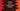# Python program to print a right angled triangle## Python 3 program to print a right angle triangle :

In this tutorial, we will learn how to print one right angle triangle using python 3. A triangle is called a right angle triangle if it’s one angle is of_ 90 degree_ or right angle.

The other two angles depend on the side length of the triangle. If the sides making the right angle are equal or if the triangle is an_ isosceles triangle, the other two angles will always _45 degrees. If the sides are not equal, then the other two angles will be different.

In this tutorial, we will create one_ isosceles right angle triangle, i.e. one angle is right angle and the other two angles are _45 degrees.

Printing a right angle triangle is the easiest task than printing any other types of triangle programmatically. In this blog post, I will show you step by step on solving this problem programmatically.

The output will look like as below :

``````1
2 2
3 3 3
4 4 4 4
5 5 5 5 5
6 6 6 6 6 6``````

As you can see, we are actually printing rows and columns here. By using loops, we can achieve results like this. We will run two loops. One for row and one for columns. For the first row, we need to print one column, two columns for the second row, three for the third row etc.

You can use any loop (for or while) you want. In this example, we will use ‘for’ loop. The outer loop will run for 6 times in the example. Let’s say it runs for i from_ 1 to 6._ The inner loop will be used to print the numbers: it will run for 1 to i.

For i =1 for outer loop, j will run for 1 for inner loop _ For i = 2 for outer loop, j will run for 1,2 for inner loop_ _ For i = 3 for outer loop, j will run for 1,2,3 for inner loop_ _ For i = 4 for outer loop, j will run for 1,2,3,4 for inner loop_ _ For i = 5 for outer loop, j will run for 1,2,3,4,5 for inner loop_ _ For i = 6 for outer loop, j will run for 1,2,3,4,5,6 for inner loop_

For each time the inner loop runs, we will print the value of ‘i’. So, for i = 1, it will print only 1 , for_ i = 2 ,_ it will print only 2 etc.

Also, the inner loop will run for ‘i’ amount of time. For the first row, it will run for one time, two times for the second row, three for the third row etc. On each iteration of the inner loop, we are printing the character.

### Python Program :

``````#example 1
height = int(input("Enter the height of the triangle :"))
for i in range(1,height+1):
for j in range(1,i+1):
print(str(i)+" ", end='')
print()``````

### Output:

Above program can be changed to print any type of right angle triangle. e.g. you can print one right angle triangle with the first row as_ ‘1’,_ second row as_ ‘1,2’, third row as _‘1,2,3’ etc.

Or you can use any other character to print the triangle like we are explaining below :

### Python program to print a right angle triangle using star (*) or any character :

We can modify the above program to print this triangle with any character we want :

``````#example 2
height = int(input("Enter the height of the triangle : "))
c = str(input("Enter the character you want to print the triangle : "))
for i in range(1,height+1):
for j in range(1,i+1):
print(c+" ", end='')
print()``````

### Sample Outputs :

As you can see that we can print triangles with any character that we want. Try to run the above program and drop one comment below if you have any queries.